# World of TrianglesPage 1

#### WATCH ALL SLIDES

Slide 1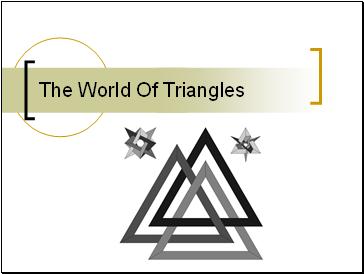The World Of Triangles

Slide 2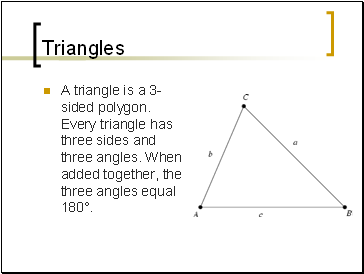## Triangles

A triangle is a 3-sided polygon. Every triangle has three sides and three angles. When added together, the three angles equal 180°.

Slide 3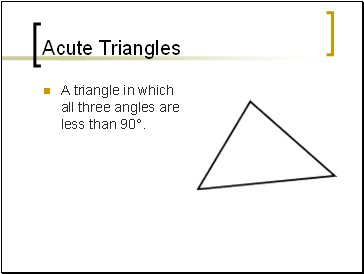## Acute Triangles

A triangle in which all three angles are less than 90°.

Slide 4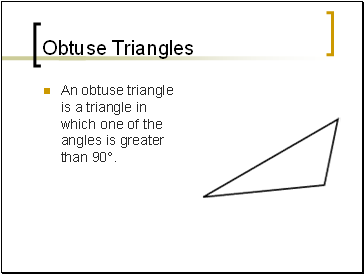## Obtuse Triangles

An obtuse triangle is a triangle in which one of the angles is greater than 90°.

Slide 5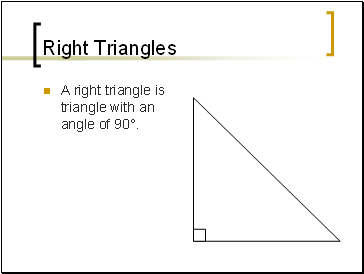## Right Triangles

A right triangle is triangle with an angle of 90°.

Slide 6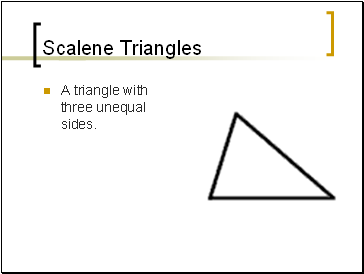## Scalene Triangles

A triangle with three unequal sides.

Slide 7## Isosceles Triangles

An isosceles triangle is a triangle with (at least) two equal sides.

Slide 8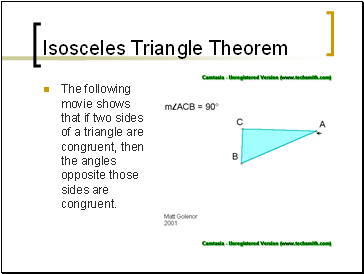## Isosceles Triangle Theorem

The following movie shows that if two sides of a triangle are congruent, then the angles opposite those sides are congruent.

Slide 9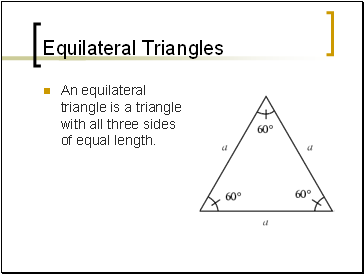## Equilateral Triangles

An equilateral triangle is a triangle with all three sides of equal length.

Slide 10Equiangular Triangles

An equiangular triangle is a triangle which has three equal angles.

Slide 11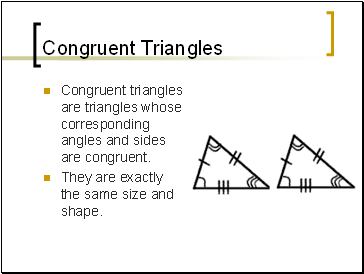## Congruent Triangles

Congruent triangles are triangles whose corresponding angles and sides are congruent.

They are exactly the same size and shape.

Slide 12## The Hypotenuse

The hypotenuse of a right triangle is the triangle's longest side, i.e., the side opposite the right angle.

Slide 13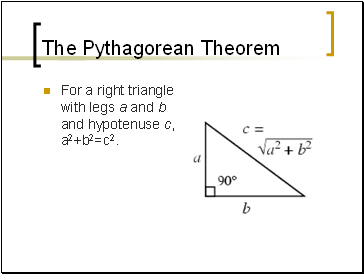## The Pythagorean Theorem

For a right triangle with legs a and b and hypotenuse c, a2+b2=c2.

Slide 14## Triangle Quiz

Please complete the following quiz that covers the information that was just presented to you.

Slide 15Go to page:
1  2